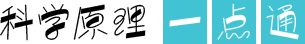# 趣味形数之正方形数

2018-08-31 07:57  来源： 新华网（1）任何一个非零偶数的平方数都可以表示为首项为4，公差为8的一串数之和的形式。即（2n）2=4+12+20+…+（8n-4）2如22=4，42=4+12，62=4+12+20，82=4+12+20+28,……（2）任何一个奇数的平方都可以表示为从1开始，然后依次是8的连续倍数的几个数之和的形式。即：（2n+1）2=1+8×1+8×2+…+8×n如12=1，32=1+8×1，42=1+8×1+8×2，52=1+8×1+8×2+8×3，……• 1

• 2

• 3

• 4

• 5